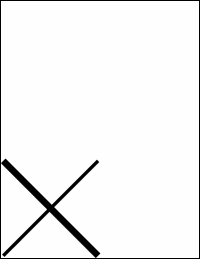Type Default Value Read Only Description Double 1 No The current line width.Notes

 The width determines the width of lines drawn using methods like AddLine and FrameRect. The width is measured in the current Units. The AddLine method creates lines centered on the points you provide. This means that if you draw a horizontal line with a width of ten, the line will extend five points above your start position and five points below it. The FrameRect method draws lines outside the rectangle you provide. So if you frame a rectangle using a width of ten, the drawn rectangle will extend ten points above, below, to the left and to the right of your specified rectangle. No drawing will fall within the rectangle.Example

 The following code adds two lines to a document. The first line has a width of ten points, and the second has a width of twenty points. ```Set theDoc = Server.CreateObject("ABCpdf11.Doc") theDoc.Width = 10 theDoc.AddLine 10, 10, 300, 300 theDoc.Width = 20 theDoc.AddLine 10, 300, 300, 10 theDoc.Save "c:\mypdfs\docwidth.pdf"```docwidth.pdf